题目描述（困难难度）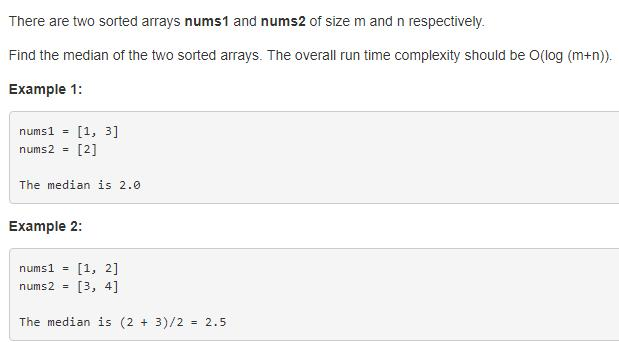解法三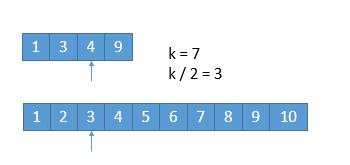A 数组中比 A [ k / 2 ] 小的数有 k / 2 - 1 个，B 数组中，B [ k / 2 ] 比 A [ k / 2 ] 小，假设 B [ k / 2 ] 前边的数字都比 A [ k / 2 ] 小，也只有 k / 2 - 1 个，所以比 A [ k / 2 ] 小的数字最多有 k / 2 - 1 + k / 2 - 1 = k - 2 个，所以 A [ k / 2 ] 最多是第 k - 1 小的数。而比 A [ k / 2 ] 小的数更不可能是第 k 小的数了，所以可以把它们排除。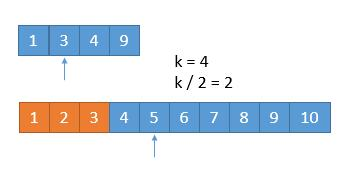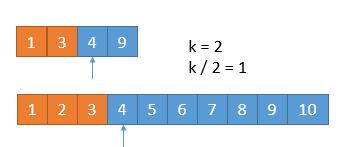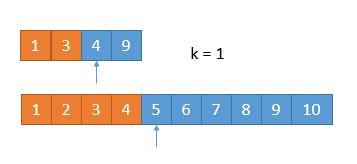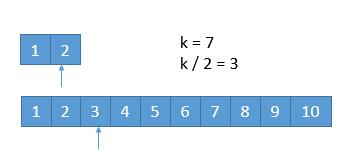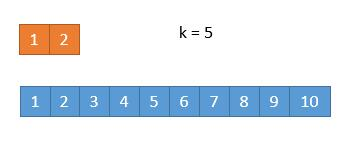解法四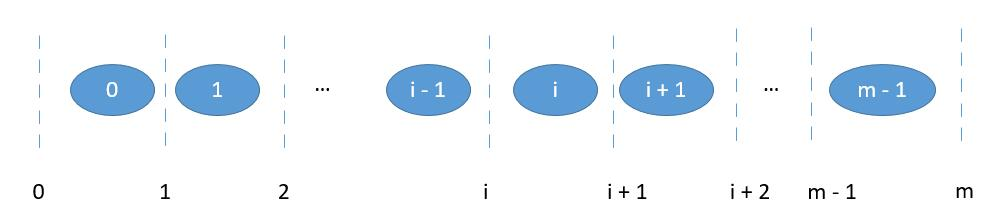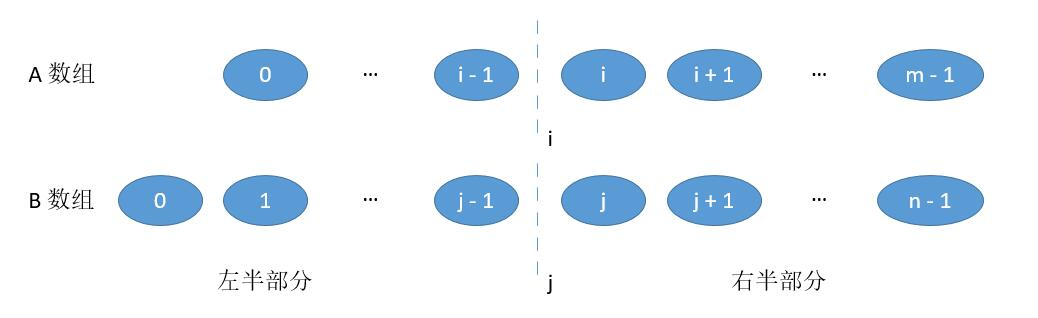• 当 A 数组和 B 数组的总长度是偶数时，如果我们能够保证

• 左半部分的长度等于右半部分

i + j = m - i + n - j , 也就是 j = ( m + n ) / 2 - i

• 左半部分最大的值小于等于右半部分最小的值 max ( A [ i - 1 ] , B [ j - 1 ]）） <= min ( A [ i ] , B [ j ]））

那么，中位数就可以表示如下

（左半部分最大值 + 右半部分最大值 ）/ 2 。

（max ( A [ i - 1 ] , B [ j - 1 ]）+ min ( A [ i ] , B [ j ]）） / 2

• 当 A 数组和 B 数组的总长度是奇数时，如果我们能够保证

• 左半部分的长度比右半部分大 1

i + j = m - i + n - j + 1也就是 j = ( m + n + 1) / 2 - i

• 左半部分最大的值小于等于右半部分最小的值 max ( A [ i - 1 ] , B [ j - 1 ]）） <= min ( A [ i ] , B [ j ]））

那么，中位数就是

左半部分最大值，也就是左半部比右半部分多出的那一个数。

max ( A [ i - 1 ] , B [ j - 1 ]）

$$m\leq n，i<m,j=(m+n+1)/2-i\geq(m+m+1)/2-i>(m+m+1)/2-m= 0$$

$$m\leq n,i>0,j=(m+n+1)/2-i\leq (n+n+1)/2-i<(n+n+1)/2=n$$

• B [ j - 1 ] > A [ i ]，并且为了不越界，要保证 j != 0，i != m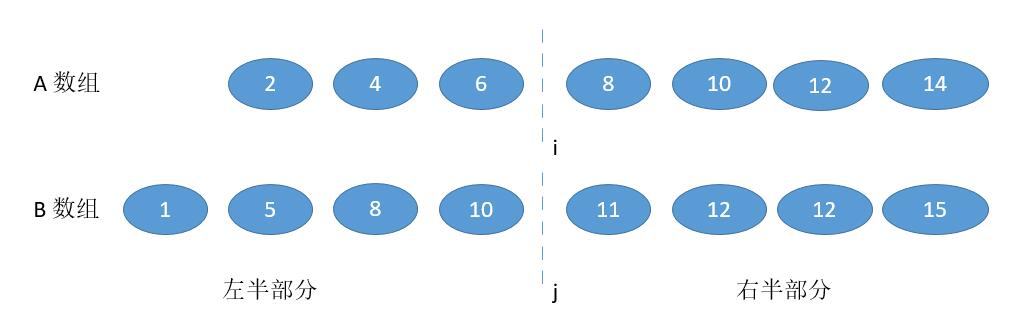此时很明显，我们需要增加 i ，为了数量的平衡还要减少 j ，幸运的是 j = ( m + n + 1) / 2 - i，i 增大，j 自然会减少。

• A [ i - 1 ] > B [ j ] ，并且为了不越界，要保证 i != 0，j != n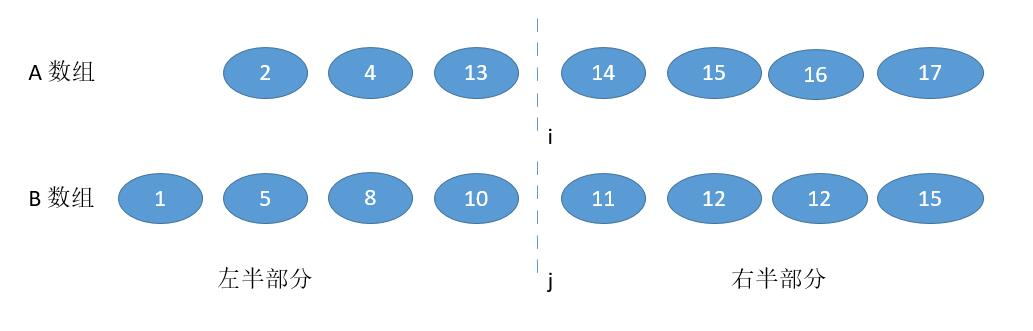此时和上边的情况相反，我们要减少 i ，增大 j 。

• 当 i = 0 , 或者 j = 0 ，也就是切在了最前边。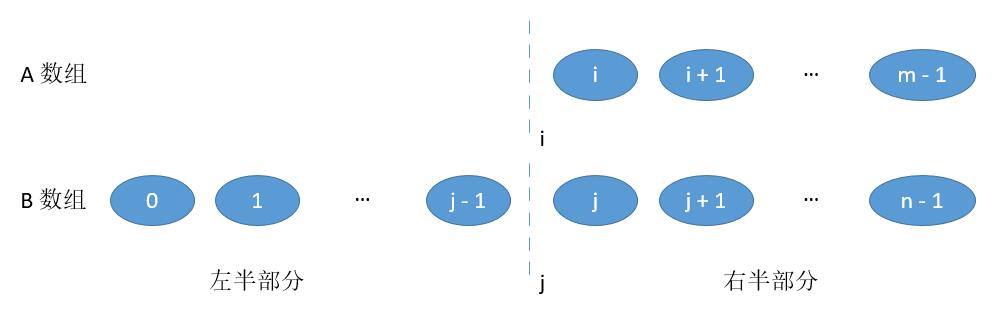此时左半部分当 j = 0 时，最大的值就是 A [ i - 1 ] ；当 i = 0 时 最大的值就是 B [ j - 1] 。右半部分最小值和之前一样。

• 当 i = m 或者 j = n ，也就是切在了最后边。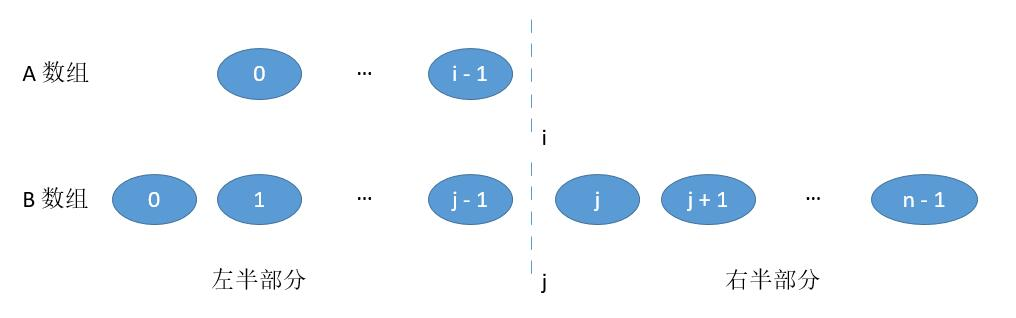此时左半部分最大值和之前一样。右半部分当 j = n 时，最小值就是 A [ i ] ；当 i = m 时，最小值就是B [ j ] 。

所有的思路都理清了，最后一个问题，增加 i 的方式。当然用二分了。初始化 i 为中间的值，然后减半找中间的，减半找中间的，减半找中间的直到答案。

总结